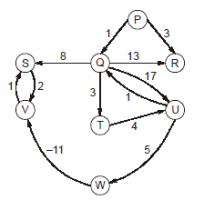# MadeEasy Test Series: Algorithms - Graph Algorithms

1.1k views

For the graph given below Dijkstra’s algorithm does not provide correct shortest path tree.Suppose a new graph that is different only in weight between Q to S is created. The number of values of edge [Q to S] that ensures that Dijkstra’s provide the correct shortest path tree where the values of edge (Q to S) ∈ [–20, 20] and ‘P’ is the source vertex are ______.

edited
0
[ -20 ,-1 ] and [ 11,20 ] are allowed .(30 values)
0

@PRIYA SHANKAR  what is the solution provided by made easy ?

0

As PQ is comman path, lets eleminate it.

Now, QTUW path is 12. But, inorder that I select the next relaxing edge as WV, QS path must be greater than 12.

So, [13 20] is one valid range.

=========================

Next, consider min shortest path in original diagram QTUWVS. Path length is 2.

Now, if I use QS as 2, still it would give me one of the correct shortest paths. If QS is less than 2, still it would work.

So, other range is [-20 2].

===========================

So, total 31 values.

Lots of corner cases : although I tried to explain in the following way :

This problem involves many situations where two same minimum distance nodes occur while running the Dijkstra algorithm.

There are two critical distances $P -> S \text { and } P -> V$

Assume QS edge cost as = \begin{align*} x \end{align*}

From direct observation we can see that shortest path from P to S can be 3 or less and shortest distance from P to V can be 2 or less. ( can go to negative ) ( from P distances 3 and 2 exist via W to S and V respectively )

Well, if we allow large negative value of edge cost of QS then S and V also will be relaxed at some negative distance away from P. This is fine according to our condition.Let's start using negative values of x starting from -20.

If we use the two constraint imposed on $\text{max} \text{ dist(P,V)}$ and $\text{max} \text{ dist(P,S)}$ then;

\begin{align*} &\text{ dist(P,V)} \leq 2 \text{ AND} \text{ dist(P,S)} \leq 3 \\ &=> 1+x+2 \leq \text{max(dist(P,V))} = 2 \ \ \text{ AND } \ \ 1+x \leq \text{max(dist(P,S))} = 3\\ &=> \text{x}\leq \text{-1} \\ \end{align*}Now in the range of \begin{align*} [-20,-1] \end{align*} if we run the Dijkstra's algorithm, then S and V will be removed early (Relaxed or no update further possible) and luckily no distance conflict with vertices S and V arise towards the end of the algorithm. They remain below their maximum limit which are 3 and 2 respectively.

Now we will start assigning positive value to the edge cost of QS. For positive values of $x$ vertices S and V will also end up being relaxed at some positive value of distances. Here we have to be carefull because, their +ve distances can not increase beyond certain limit as mentioned earlier.

Now if we don't Relax them (S and V) early in the running algorithm (or, retain their updating possibility towards the end) , then we must also have to keep them (without the chance of being relaxed) till the end such that their distances can be correctly updated (to 3 and 2) using W or via W.

How can we keep them (vertices S and V ) in the algorithm without relaxing early.

Note that we can not intentionally keep them (S and V) unused (unrelaxed) nodes if in a particular step their distance become the minimum.

So, the point is if some vertices are not relaxed towards the end, that means their distance value are more than the currently existing large value of distance (of not relaxed nodes).***$\textbf{}$

So, We conclude that S and V can only be relaxed after W has been relaxed.

Now we know that the shortest path from P to W is of distance 13. So, during Dijkstra's relaxing rounds of vertices, to keep both of S and V unrelaxed then;

\begin{align*} & 1+x > 13 \ \ \ \text{AND}, \ \ \ 1+x+2 > 13 \\ &=> x > 12 \end{align*}

So from

\begin{align*} x = 13 \text{ to } x = 20 \end{align*}

we will have no issue of S and V getting Relaxed before W.

Final Ranges of edge cost of QS is \begin{align*} [-20,-1] \ \ \text{ and } [13,20] \\ \end{align*}

***PS$\textbf{}$: [ little bit exception to this statement : for x = 10,11.etc.  S is not relaxed although its distance value (11,12 etc) is less than 18 of U, because at that time T is also present having the value 4, so T was selected ]

$[\textbf{2}]$ : I haved assumed one criteria: when two or more vertices with same distance occur while running the Dijkstra's algorith ,it could select any one of them for relaxing.

edited by
1
I explained the same above in my posted pic :P :P

But nobody gave a dammn! uhh!!
0
May be Your pic is not readable.  But as soon as i found same i gave +1
0
...........

## Related questions

1 vote
1
1 vote
I read that the space complexity of Dijasktra is $O(V^2)$ . (http://igraph.wikidot.com/algorithm-space-time-complexity) But how ????